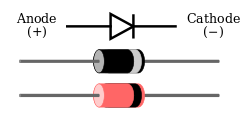Diodes allow electricity to flow in only one direction. The arrow of the circuit symbol shows the direction in which the current can flow through the diode. Diodes are very much like a one way valve, if fact early diodes where actually called valves.When a power source is connected to a diode, positive source on the anode and the negative source on the cathode the diode becomes a conductor and allows current to flow through the diode. When a diode is connected in this manor the voltage passing through the diode is called the forward bias or sometimes referred to as the forward voltage.

If you reverse the power source by connecting the positive source on the cathode and the negative source on the anode the current does not flow and effectively the diode becomes an insulator. Voltage in the diode in this direction is called the reverse bias or sometimes referred to as reverse voltage.

Forward voltage drop: Electricity is used up pushing its way through the diode, this means that there is a small voltage across a conducting diode, it is called the forward voltage drop and is about 0.7V for all normal diodes which are made from silicon. The forward voltage drop of a diode is almost constant whatever the current passing through the diode.

Reverse Voltage: When a reverse voltage is applied a working diode does not conduct, but all diodes do leak a very tiny amount of current this is just a few μA or less. This can be ignored in most circuits because it will be much smaller than the current flowing in the forward direction. However, all diodes have a maximum reverse voltage (usually 50V or more) and if this is exceeded the diode will fail and pass a large amount of current in the reverse direction.

Ordinary diodes can be split into two types: Signal diodes which pass small currents of 100mA or less and Rectifier diodes which can pass large currents. In addition there are LED's and Zener diodes.

Signal diodes (small current): Signal diodes are used to process information, electrical signals in circuits, so they are only required to pass small currents of up to 100mA. Signal diodes are also used to protect transistors and ICs in circuits. General purpose signal diodes are made from silicon and have a forward voltage drop of 0.7V.

Germanium diodes have a lower forward voltage drop of 0.2V and this makes them suitable to use in radio circuits as detectors which extract the audio signal from the weak radio signal.

Rectifier diodes (large current): Rectifier diodes are used in power supplies to convert alternating current (AC) to direct current (DC), this process is called rectification. They are also used in many other parts of a circuit where a large current must pass through the diode and to stop current from flowing the wrong way in a circuit.

All rectifier diodes are made from silicon and have a forward voltage drop of 0.7V. The table below shows maximum current and maximum reverse voltage for some popular rectifier diodes. The 1N4001 is suitable for most low voltage circuits with a current of less than 1A.

 Diode Max Reverse Voltage Maximum Current 1N4001 50V 1A 1N4002 100V 1A 1N4003 200V 1A 1N4004 400V 1A 1N4006 800V 1A 1N4007 1000V 1A